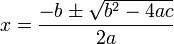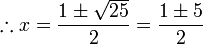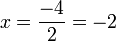For the quadratic equation ax2 + bx + c = 0, the real solutions are given by the quadratic formula:$x=\frac{-b\pm\sqrt{b^2-4ac}}{2a}$

##  Discriminant

The discriminant Δ in the quadratic equation is given by:

Δ = b2 − 4ac

The discriminant can be used to provide information about the number of solutions to a quadratic equation:

• if Δ > 0, there are two unique real solutions
• if Δ = 0, there is one unique real solution
• if Δ < 0, there are no unique real solutions.

##  Example

Suppose one needed to find solutions for the equation x2 − 6 = x.

First we rearrange the equation to make it equal zero:

x2 − 6 = x$\therefore x^2-x-6=0$$x=\frac{-b\pm\sqrt{b^2-4ac}}{2a}$$a=1, b=-1, c=-6 \therefore x=\frac{-(-1)\pm\sqrt{(-1)^2-4(1)(-6)}}{2(1)}$$\therefore x=\frac{1\pm\sqrt{25}}{2}=\frac{1\pm5}{2}$$\therefore$$x=\frac{6}{2}=3$ or$x=\frac{-4}{2}=-2$.